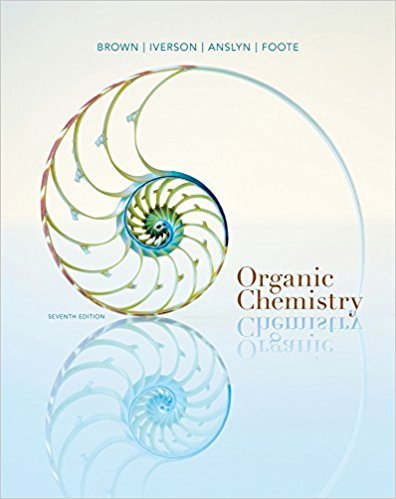×
Get Full Access to Organic Chemistry - 7 Edition - Chapter 2 - Problem 2.64
Get Full Access to Organic Chemistry - 7 Edition - Chapter 2 - Problem 2.64

×

# Following is the structural formula and a ball-and-stick model of cholestanol. TheISBN: 9781133952848 483

## Solution for problem 2.64 Chapter 2

Organic Chemistry | 7th Edition

• Textbook Solutions
• 2901 Step-by-step solutions solved by professors and subject experts
• Get 24/7 help from StudySoup virtual teaching assistantsOrganic Chemistry | 7th Edition

4 5 1 342 Reviews
11
3
Problem 2.64

Following is the structural formula and a ball-and-stick model of cholestanol. The onlydifference between this compound and cholesterol (Section 26.4) is that cholesterol hasa carbon-carbon double bond in ring B. (a) Describe the conformation of rings A, B, C, and D in cholestanol.(b) Is the hydroxyl group on ring A axial or equatorial?(c) Consider the methyl group at the junction of rings A and B. Is it axial or equatorialto ring A? Is it axial or equatorial to ring B?(d) Is the methyl group at the junction of rings C and D axial or equatorial to ring C?

Step-by-Step Solution:
Step 1 of 3

Chemistry Skills and Reasoning 1 Week 10 Chapter 13: Gases - Gases uniformly ﬁll the container, mixes completely with any other gas, and exerts pressure on its surroundings - Barometer is a device used to measure atmospheric pressure - Boyle”s Law: pressure and volume are inversely related - P1V1 = P2V2 - Charles’ Law: volume and temperature are directly related - V1T1 = V2T2 - Avogadro’s Law: volume and number of moles are directly related - V1n2 = V2n1 - Ideal Gas Law: PV = NRT - the units must always be atm, L, mol, and K - The total pressure exerted is the sum of the pressure that each gas would exert if it were alone - The pressure of the gas is affected by the number of moles of particles present, the pressure is independent of the nature of the particles - Postulates of the Kinetic Molecular Theory - Gases consist o f tiny particles (atoms or molecules - the particles are so small, compared with the distances between them that the volume (size) of the individual particles can be assumed to be negligible (zero) - The particles are in constant random motion, colliding with the walls of the container. These collisions with the walls cause the pressure exerted by the gas - The particles are assumed not to attract or to repel each other - The average kinetic energy of the gas particles is directly proportion to the Kelvin temperature of the gas The whole thing to take away from this chapter is the math and the theory behind it, that is

Step 2 of 3

Step 3 of 3

##### ISBN: 9781133952848

The answer to “Following is the structural formula and a ball-and-stick model of cholestanol. The onlydifference between this compound and cholesterol (Section 26.4) is that cholesterol hasa carbon-carbon double bond in ring B. (a) Describe the conformation of rings A, B, C, and D in cholestanol.(b) Is the hydroxyl group on ring A axial or equatorial?(c) Consider the methyl group at the junction of rings A and B. Is it axial or equatorialto ring A? Is it axial or equatorial to ring B?(d) Is the methyl group at the junction of rings C and D axial or equatorial to ring C?” is broken down into a number of easy to follow steps, and 98 words. Organic Chemistry was written by and is associated to the ISBN: 9781133952848. The full step-by-step solution to problem: 2.64 from chapter: 2 was answered by , our top Chemistry solution expert on 03/16/18, 05:02PM. Since the solution to 2.64 from 2 chapter was answered, more than 254 students have viewed the full step-by-step answer. This full solution covers the following key subjects: . This expansive textbook survival guide covers 24 chapters, and 1131 solutions. This textbook survival guide was created for the textbook: Organic Chemistry, edition: 7.

Unlock Textbook Solution